## RS Aggarwal Class 7 Solutions Chapter 8 Ratio and Proportion Ex 8A

These Solutions are part of RS Aggarwal Solutions Class 7. Here we have given RS Aggarwal Solutions Class 7 Chapter 8 Ratio and Proportion Ex 8A.

Other Exercises

Question 1.
Solution:
(i) 24 : 40
HCF of 24, 40 = 8
24 : 40 = 24 ÷ 8 : 40 ÷ 8 = 3 : 5 (Dividing by 8)
(ii) 13.5 : 15 or 135 : 150
HCF of 135 and 150 = 15
135 ÷ 15 : 150 ÷ 15 (Dividing by 15)
= 9 : 10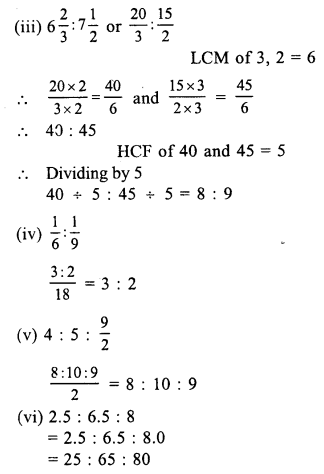HCF of 25, 65, 80 = 5
Dividing by 5,
5 : 13 : 16

Question 2.
Solution:
(i) 75 paise : 3 rupees = 75 paise : 300 paise
(converting into same kind)
HCF of 75, 300 = 75
75 : 300 = 75 ÷ 75 : 300 ÷ 75 (Dividing by 75) = 1 : 4
(ii) 1 m 5 cm : 63 cm = 105 cm : 63 cm
(converting into same kind)
HCF of 105 and 63 = 21
105 ÷ 21 : 63 ÷ 21 (Dividing by 21)
= 5 : 3
(iii) 1 hour 5 minutes : 45 minutes = 65 minutes : 45 minutes
(converting into minutes)
13 : 9 (dividing by 5)
= 13 : 9
(iv) 8 months : 1 year = 8 months : 12 months
(converting into the same kind)
HCF of 8 and 12 = 4
Dividing by 4
8 ÷ 4 : 12 ÷ 4
= 2 : 3
(v) 2 kg 250 g : 3 kg = 2250 g : 3000 g (converting into the same kind)
HCF of 2250 and 3000 = 750
Dividing by 750,
2250 ÷ 750 : 3000 ÷ 750 = 3 : 4
(vi) 1 km : 750 m = 1000 m : 750 m
(converting into metre)
= 4 : 3 (dividing by 250)
= 4 : 3

Question 3.
Solution:Question 4.
Solution:
A : B = 5 : 8, B : C = 16 : 25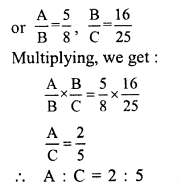Question 5.
Solution:
A : B = 3 : 5, B : C = 10 : 13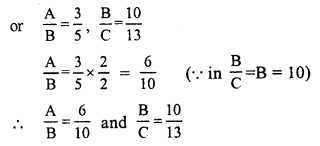A : B : C = 6 : 10 : 13

Question 6.
Solution:
A : B = 5 : 6 and B : C = 4 : 7Question 7.
Solution:
Total amount = Rs. 360
Sum of ratios = 7 + 8 = 15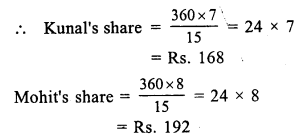Question 8.
Solution:
Total amount = Rs. 880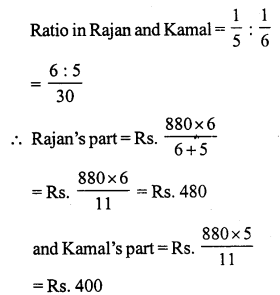Question 9.
Solution:
Total amount = Rs. 5600
Ratio in A : B : C = 1 : 3 : 4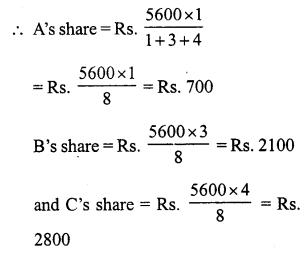Question 10.
Solution:
Let x be added to each term Then
9 + x : 16 + x = 2 : 3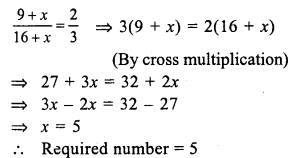Question 11.
Solution:
Let x be subtracted from each term Then
(17 – x) : (33 – x) = 7 : 15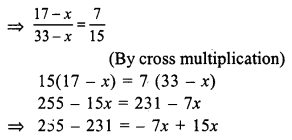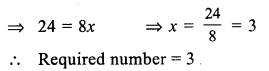Question 12.
Solution:
Ratio in two numbers = 7 : 11
Let first number = 7x
Then second number = 11x
Then adding 7 to each number, the ratio is 2 : 3
$$\frac { 7x + 7 }{ 11x + 7 }$$ = $$\frac { 2 }{ 3 }$$
By cross multiplying:
3 (7x + 7) = 2 (11x + 7)
⇒ 21x + 21 = 22x + 14
⇒ 21 – 14 = 22x – 21x
⇒ x = 7
First number = 7x = 7 x 7 = 49
and second number = 11x = 11 x 7 = 77
Hence numbers are 49, 77

Question 13.
Solution:
The ratio in two numbers = 5 : 9
Let the first number = 5x
Then second number = 9x
By subtracting 3 from each number the ratio is 1 : 2
$$\frac { 5x – 3 }{ 9x – 3 }$$ = $$\frac { 1 }{ 2 }$$
By cross multiplication,
2 (5x – 3) = 1 (9x – 3)
⇒ 10x – 6 = 9x – 3
⇒ 10x – 9x = -3 + 6
⇒ x = 3
First number = 5x = 5 x 3 = 15
and second .number = 9x = 9 x 3 = 27
Hence numbers are 15, 27

Question 14.
Solution:
Ratio in two numbers = 3 : 4
LCM = 180
Let first number = 3x
Then second number = 4x
Now LCM = 3 x 4 x x = 12x
12x = 180
⇒ x = 15
Numbers will be 3 x 15 = 45 and 4 x 15 = 60

Question 15.
Solution:
Ratio in present ages of A and B = 8 : 3
Let A’s age = 8x
Then B’s age = 3x
6 years hence,
A’s age will be = 8x + 6
and B’s will be = 3x + 6
$$\frac { 8x + 6 }{ 3x + 6 }$$ = $$\frac { 9 }{ 4 }$$
(By cross multiplication)
4 (8x + 6) = 9 (3x + 6)
⇒ 32x + 24 = 27x + 54
⇒ 32x – 27x = 54 – 24
⇒ 5x = 30
⇒ x = 6
A’s present age = 8x = 8 x 6 = 48 years
and B’s age = 3x = 3 x 6 = 18 years

Question 16.
Solution:
Ratio in copper and zinc = 9 : 5
Let alloy = x gm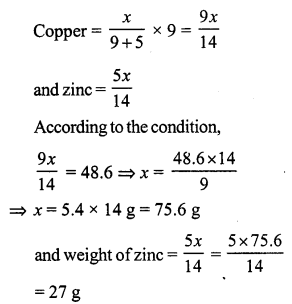Question 17.
Solution:
Ratio in boys and girls = 8 : 3
and total number of girls = 375
Let number of boys = 8x
Then number of girls = 3x
3x = 375
⇒ x = 125
Number of boys = 8x = 8 x 125 = 1000

Question 18.
Solution:
Ratio in income and savings = 11 : 2
Let income = 11x
Then savings = 2x
But savings = Rs. 2500
2x = 2500
⇒ x = 1250
Then income = 1250 x 11 = Rs. 13750
and expenditure = Total income – savings = 13750 – 2500 = Rs. 11250

Question 19.
Solution:
Total amount = Rs. 750
Ratio in rupee, 50 P and 25 P coins =5 : 8 : 4
Let number of rupees = 5x
Number of 50 P coins = 8x
and number of 25 coins = 4x
According to the condition,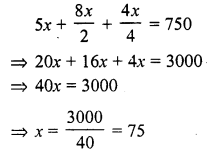Number of 1 Re coins = 5x = 5 x 75 = 375
Number of 50 P coins = 8x = 8 x 75 = 600
and number of 25 P coins = 4x = 4 x 75 = 300

Question 20.
Solution:
(4x + 5) : (3x + 11) = 13 : 17
$$\frac { 4x + 5 }{ 3x + 11 }$$ = $$\frac { 13 }{ 17 }$$
By cross multiplication,
68x + 85 = 39x + 143
⇒ 68x – 39x = 143 – 85
⇒ 29x = 58
x = 2
Hence x = 2

Question 21.
Solution:
x : y = 3 : 4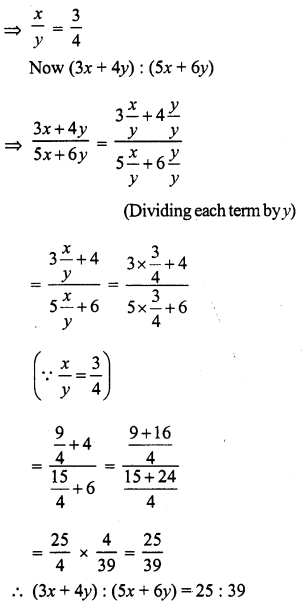Question 22.
Solution:
x : y = 6 : 11
$$\frac { x }{ y }$$ = $$\frac { 6 }{ 11 }$$
Now (8x – 3y) : (3x + 2y)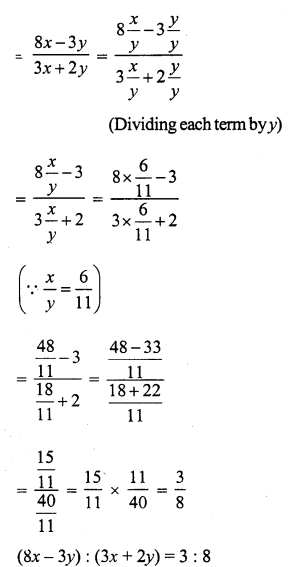Question 23.
Solution:
Sum of two numbers = 720
Ratio of two numbers = 5 : 7
Let first number = 5x
Then second number = 7x
5x + 7x = 720
⇒ 12x = 720
⇒ x = 60
First number = 5x = 5 x 60 = 300
and second number = 7x = 7 x 60 = 420

Question 24.
Solution:
(i) (5 : 6) or (7 : 9)Question 25.
Solution:
(i) (5 : 6), (8 : 9), (11 : 18)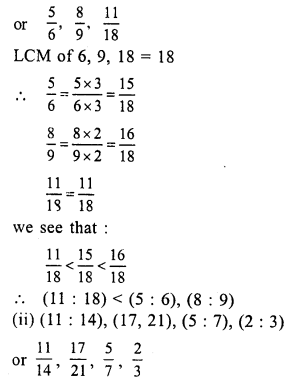Hope given RS Aggarwal Solutions Class 7 Chapter 8 Ratio and Proportion Ex 8A are helpful to complete your math homework.

If you have any doubts, please comment below. Learn Insta try to provide online math tutoring for you.